# Selina Solutions Concise Mathematics Class 6 Chapter 7: Number Line Exercise 7(B)

Selina Solutions Concise Mathematics Class 6 Chapter 7 Number Line Exercise 7(B) helps students in representing the integers on a Number Line and solving them accurately. Students, who practice Selina Solutions regularly, will become keener to learn Mathematics. Faculty at BYJU’S, formulated the solutions with the aim to help students score good marks in the academic year. This also enhances their thinking capacity while solving the textbook questions, along with boosting their confidence. Students, who have any confusion regarding the concepts, are advised to go through these solutions in detail. Avail Selina Solutions Concise Mathematics Class 6 Chapter 7 Exercise 7(B) PDF, from the links given below

## Selina Solutions Concise Mathematics Class 6 Chapter 7: Number Line Exercise 7(B) Download PDF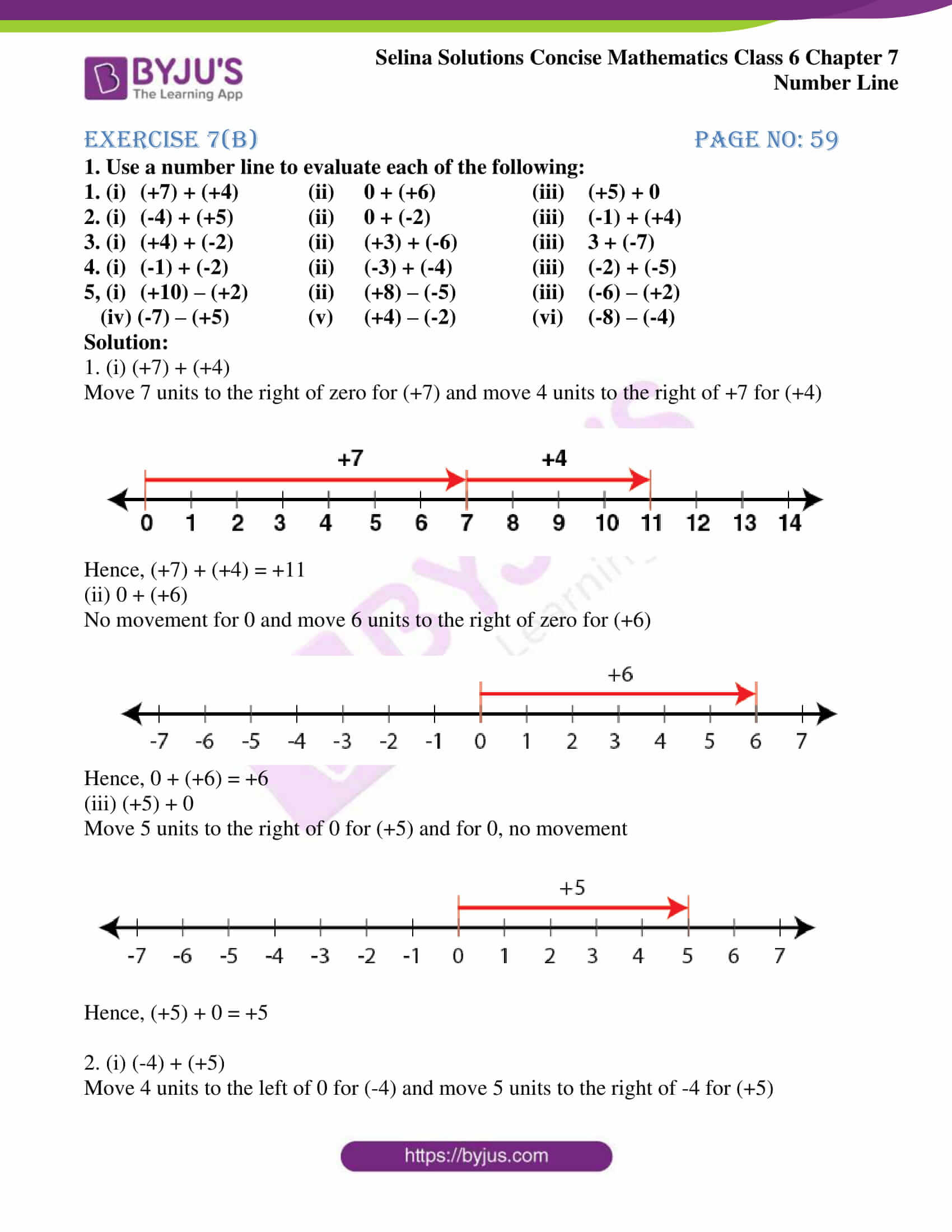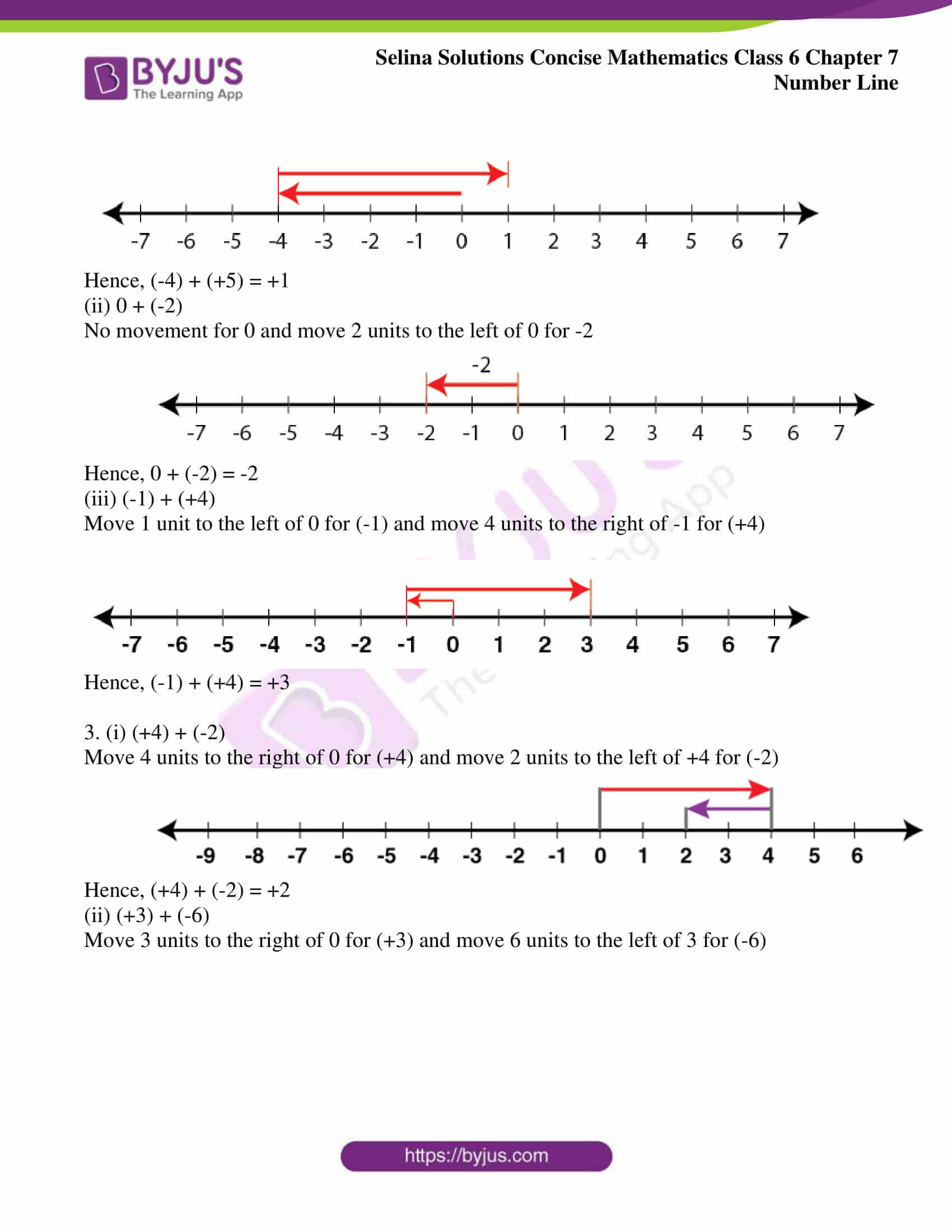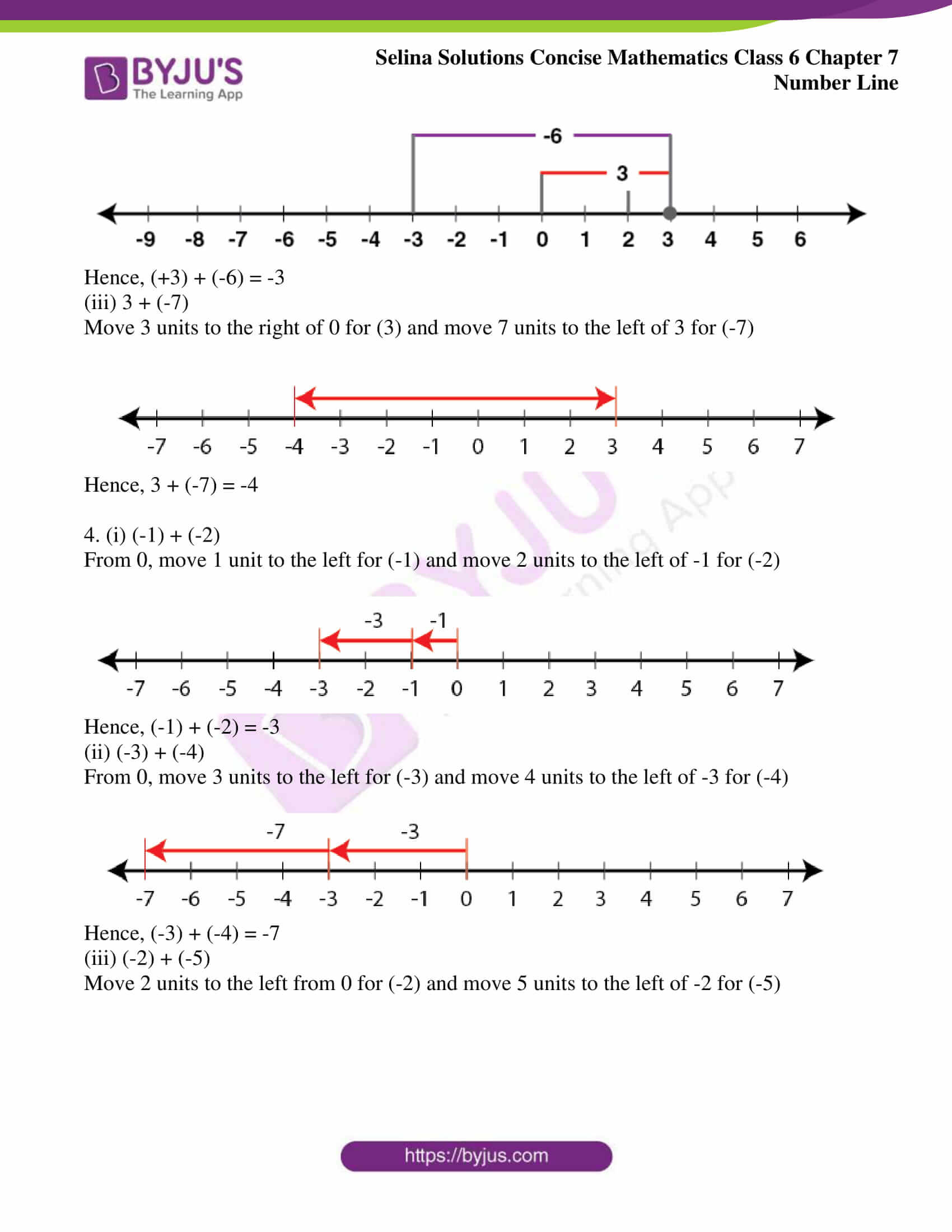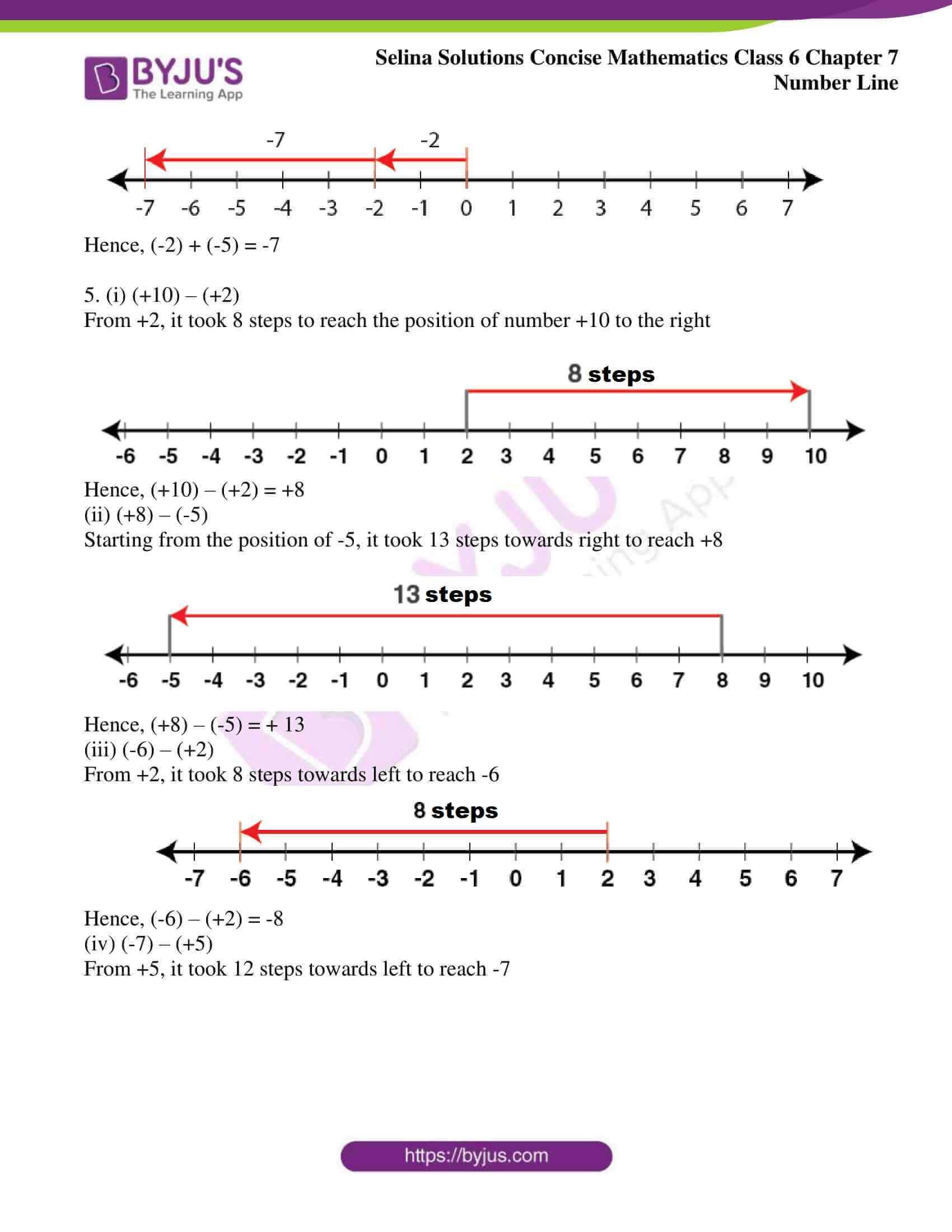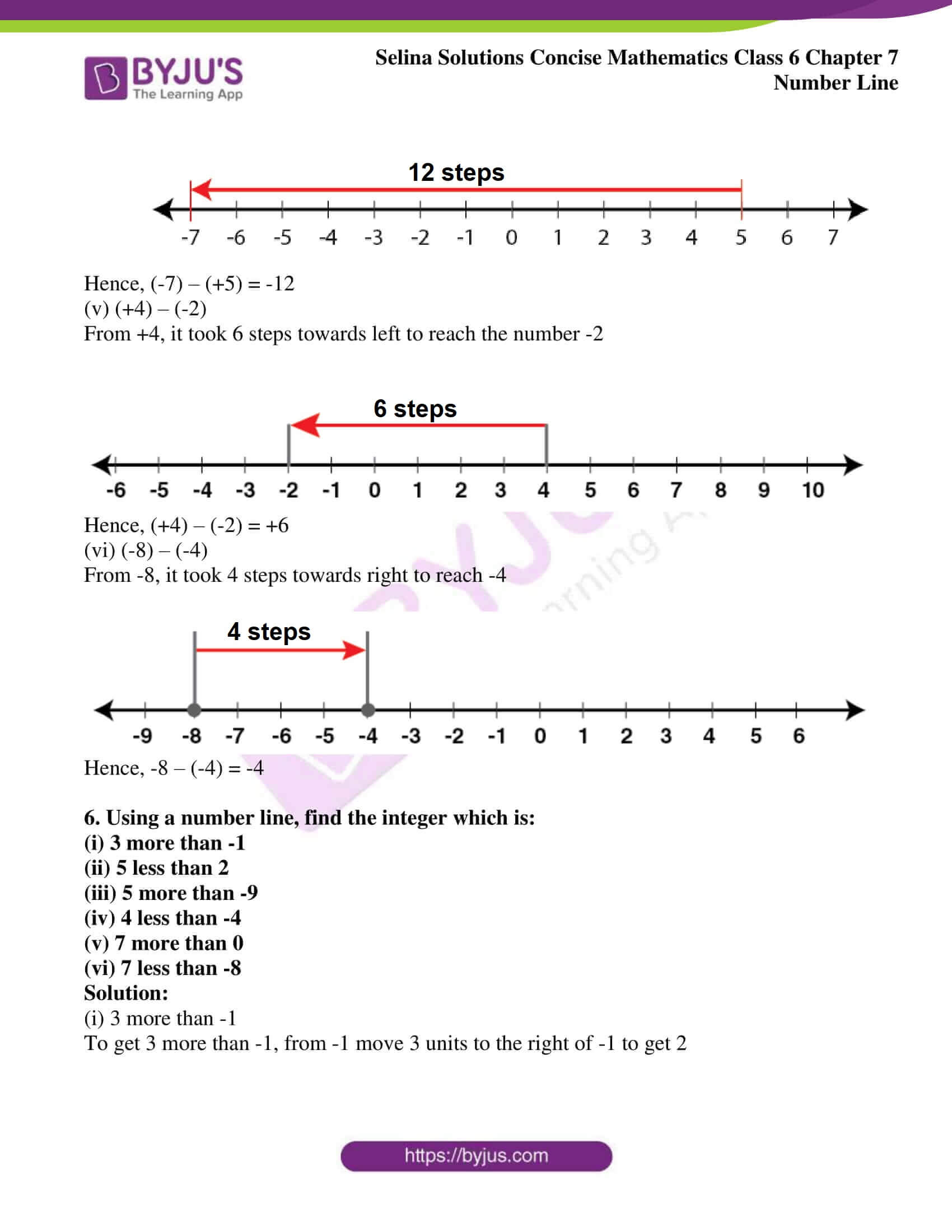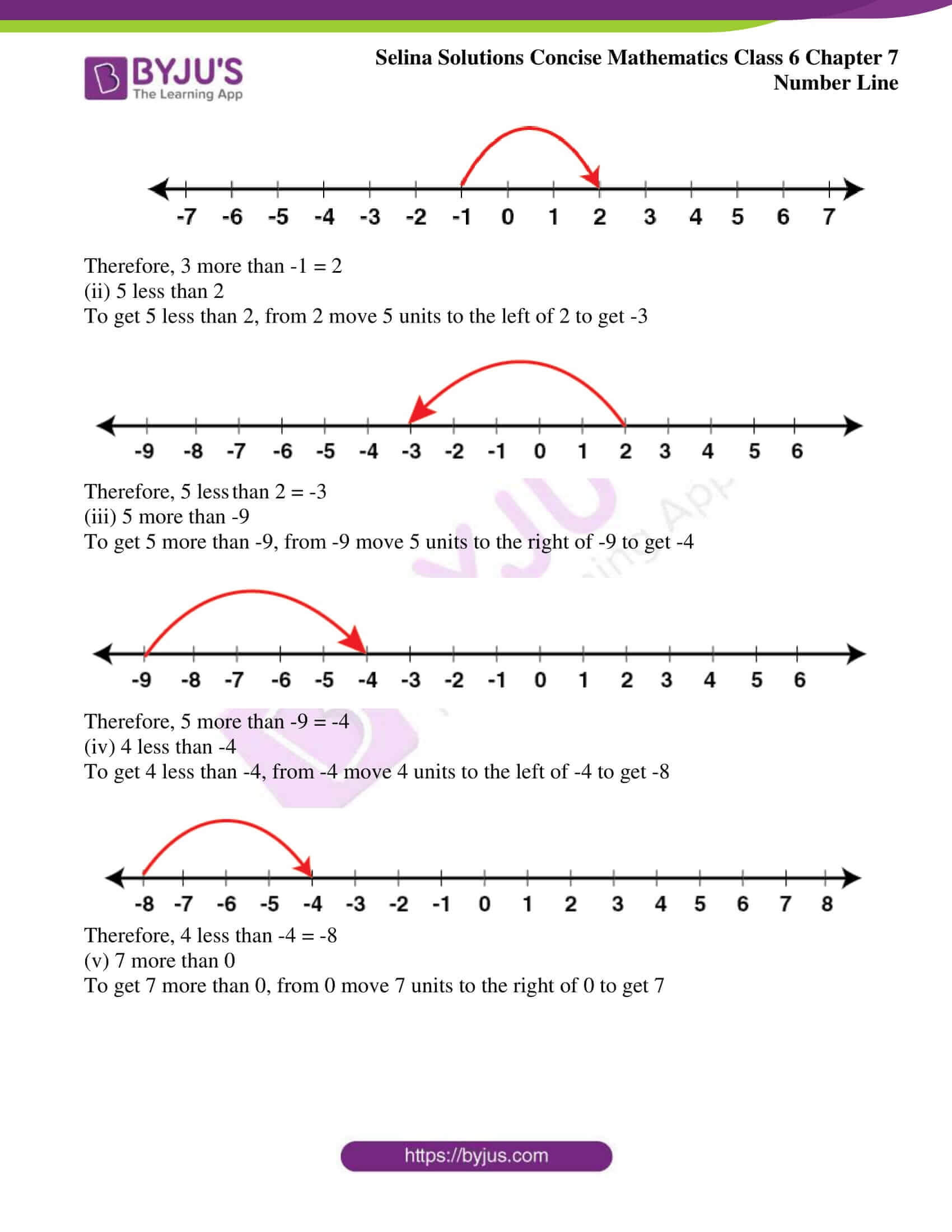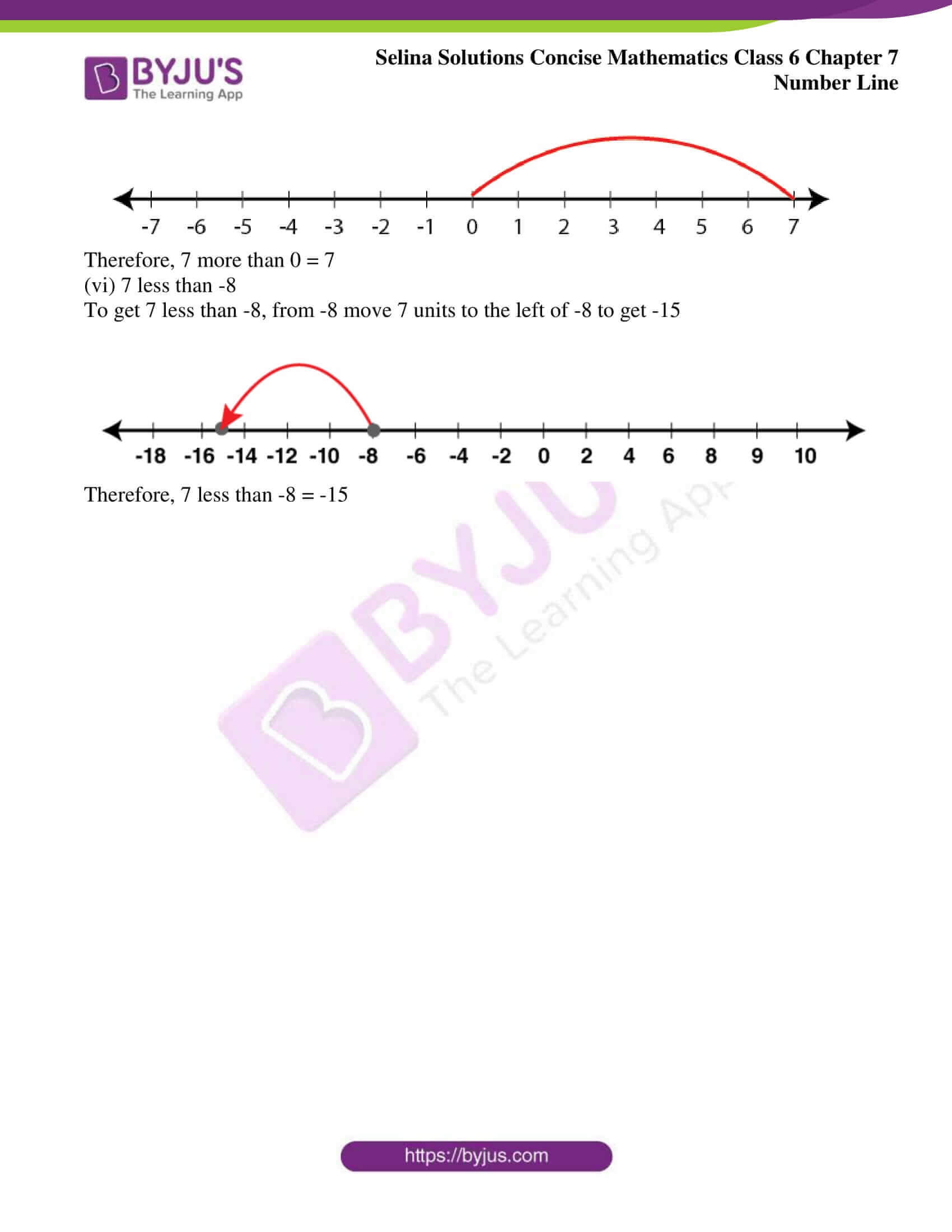### Access another exercise of Selina Solutions Concise Mathematics Class 6 Chapter 7: Number Line

Exercise 7(A) Solutions

### Access Selina Solutions Concise Mathematics Class 6 Chapter 7: Number Line Exercise 7(B)

#### Exercise 7(B) page no: 59

1. Use a number line to evaluate each of the following:

1. (i) (+7) + (+4) (ii) 0 + (+6) (iii) (+5) + 0

2. (i) (-4) + (+5) (ii) 0 + (-2) (iii) (-1) + (+4)

3. (i) (+4) + (-2) (ii) (+3) + (-6) (iii) 3 + (-7)

4. (i) (-1) + (-2) (ii) (-3) + (-4) (iii) (-2) + (-5)

5, (i) (+10) – (+2) (ii) (+8) – (-5) (iii) (-6) – (+2)

(iv) (-7) – (+5) (v) (+4) – (-2) (vi) (-8) – (-4)

Solution:

1. (i) (+7) + (+4)

Move 7 units to the right of zero for (+7) and move 4 units to the right of +7 for (+4)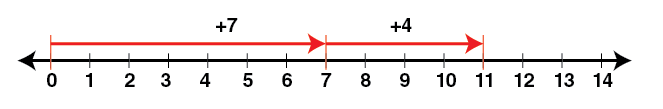Hence, (+7) + (+4) = +11

(ii) 0 + (+6)

No movement for 0 and move 6 units to the right of zero for (+6)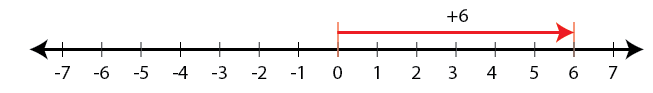Hence, 0 + (+6) = +6

(iii) (+5) + 0

Move 5 units to the right of 0 for (+5) and for 0, no movement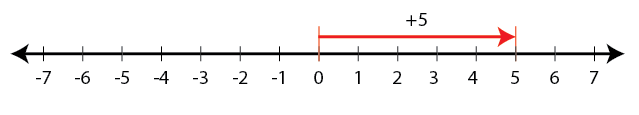Hence, (+5) + 0 = +5

2. (i) (-4) + (+5)

Move 4 units to the left of 0 for (-4) and move 5 units to the right of -4 for (+5)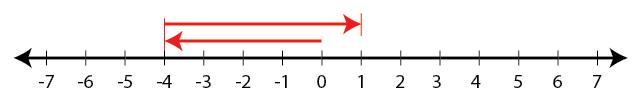Hence, (-4) + (+5) = +1

(ii) 0 + (-2)

No movement for 0 and move 2 units to the left of 0 for -2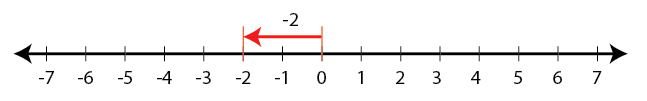Hence, 0 + (-2) = -2

(iii) (-1) + (+4)

Move 1 unit to the left of 0 for (-1) and move 4 units to the right of -1 for (+4)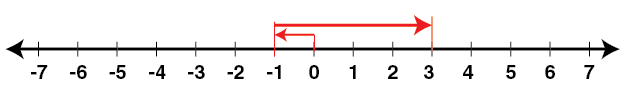Hence, (-1) + (+4) = +3

3. (i) (+4) + (-2)

Move 4 units to the right of 0 for (+4) and move 2 units to the left of +4 for (-2)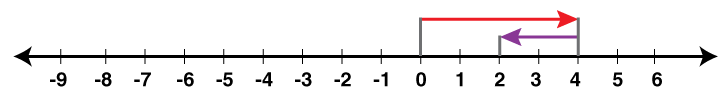Hence, (+4) + (-2) = +2

(ii) (+3) + (-6)

Move 3 units to the right of 0 for (+3) and move 6 units to the left of 3 for (-6)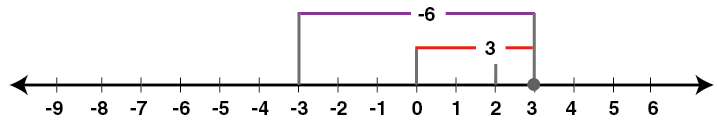Hence, (+3) + (-6) = -3

(iii) 3 + (-7)

Move 3 units to the right of 0 for (3) and move 7 units to the left of 3 for (-7)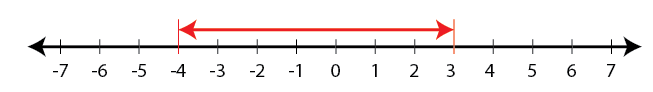Hence, 3 + (-7) = -4

4. (i) (-1) + (-2)

From 0, move 1 unit to the left for (-1) and move 2 units to the left of -1 for (-2)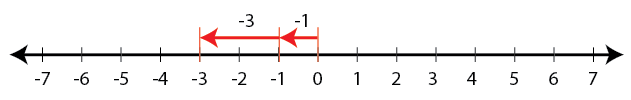Hence, (-1) + (-2) = -3

(ii) (-3) + (-4)

From 0, move 3 units to the left for (-3) and move 4 units to the left of -3 for (-4)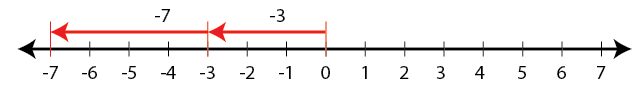Hence, (-3) + (-4) = -7

(iii) (-2) + (-5)

Move 2 units to the left from 0 for (-2) and move 5 units to the left of -2 for (-5)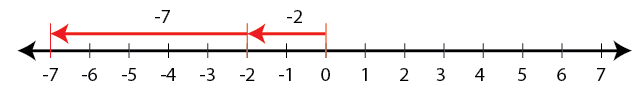Hence, (-2) + (-5) = -7

5. (i) (+10) – (+2)

From +2, it took 8 steps to reach the position of number +10 to the right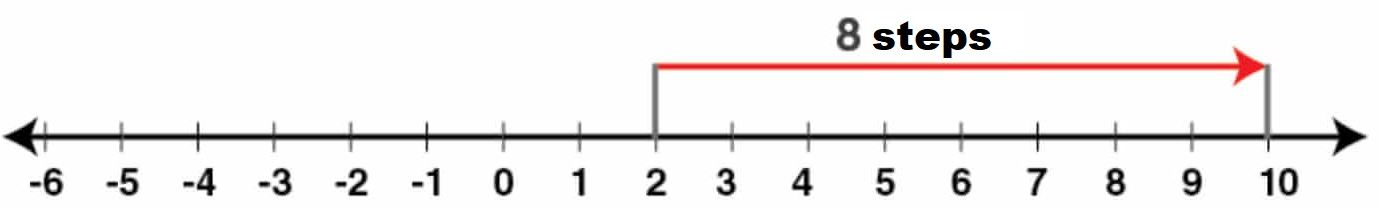Hence, (+10) – (+2) = +8

(ii) (+8) – (-5)

Starting from the position of -5, it took 13 steps towards right to reach +8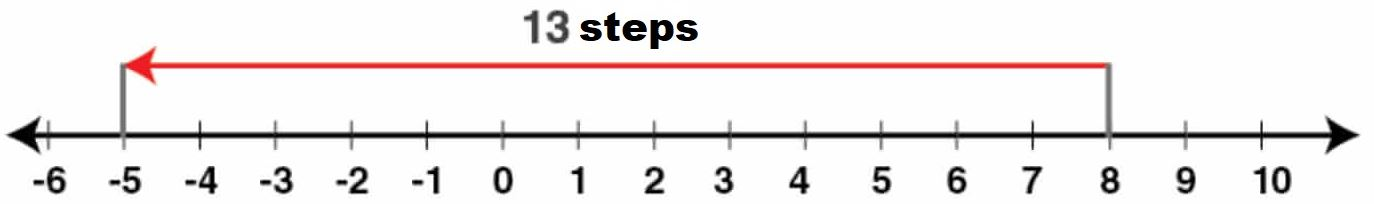Hence, (+8) – (-5) = + 13

(iii) (-6) – (+2)

From +2, it took 8 steps towards left to reach -6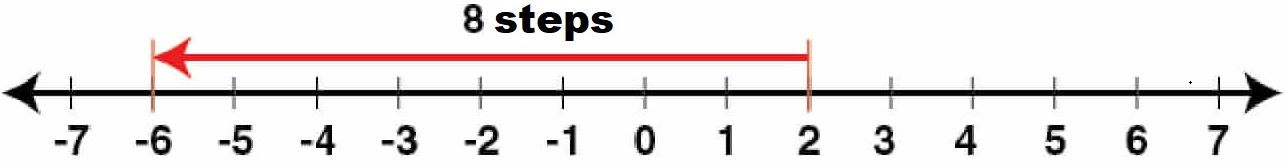Hence, (-6) – (+2) = -8

(iv) (-7) – (+5)

From +5, it took 12 steps towards left to reach -7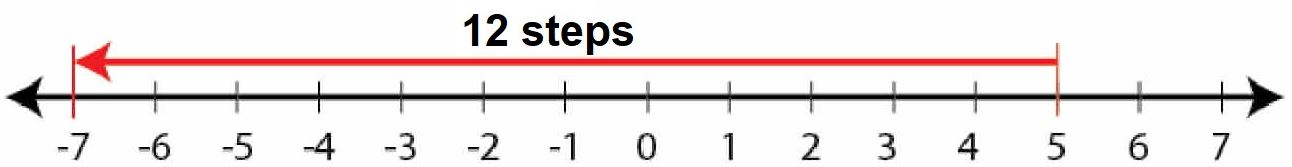Hence, (-7) – (+5) = -12

(v) (+4) – (-2)

From +4, it took 6 steps towards left to reach the number -2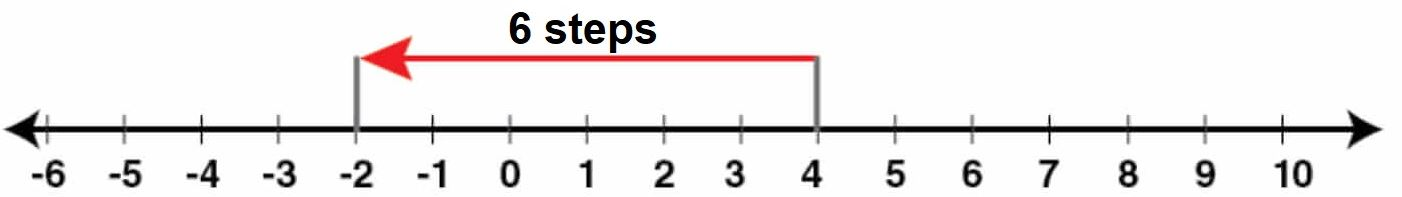Hence, (+4) – (-2) = +6

(vi) (-8) – (-4)

From -8, it took 4 steps towards right to reach -4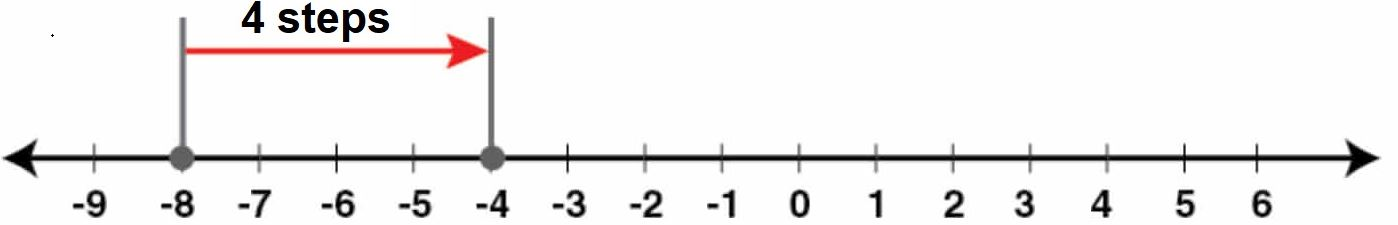Hence, -8 – (-4) = -4

6. Using a number line, find the integer which is:

(i) 3 more than -1

(ii) 5 less than 2

(iii) 5 more than -9

(iv) 4 less than -4

(v) 7 more than 0

(vi) 7 less than -8

Solution:

(i) 3 more than -1

To get 3 more than -1, from -1 move 3 units to the right of -1 to get 2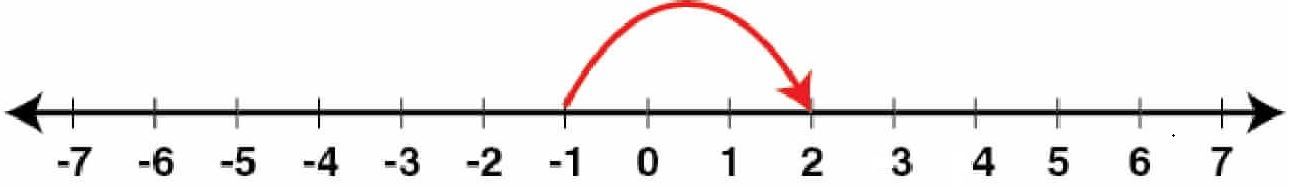Therefore, 3 more than -1 = 2

(ii) 5 less than 2

To get 5 less than 2, from 2 move 5 units to the left of 2 to get -3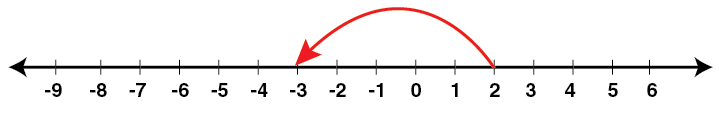Therefore, 5 less than 2 = -3

(iii) 5 more than -9

To get 5 more than -9, from -9 move 5 units to the right of -9 to get -4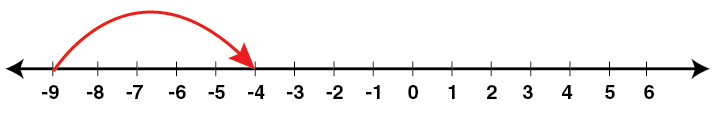Therefore, 5 more than -9 = -4

(iv) 4 less than -4

To get 4 less than -4, from -4 move 4 units to the left of -4 to get -8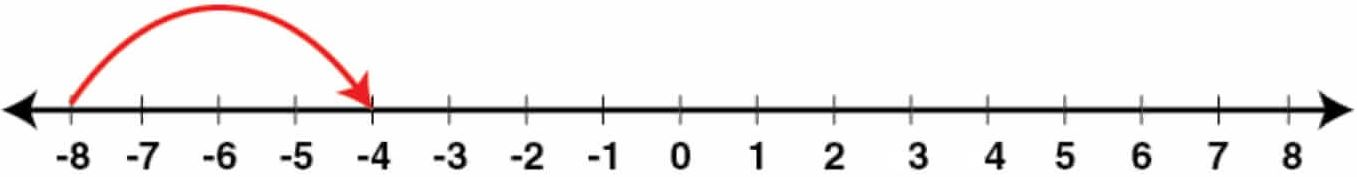Therefore, 4 less than -4 = -8

(v) 7 more than 0

To get 7 more than 0, from 0 move 7 units to the right of 0 to get 7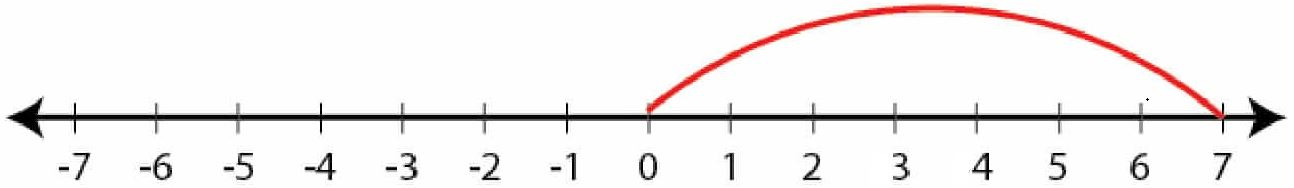Therefore, 7 more than 0 = 7

(vi) 7 less than -8

To get 7 less than -8, from -8 move 7 units to the left of -8 to get -15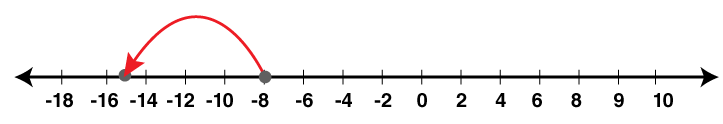Therefore, 7 less than -8 = -15